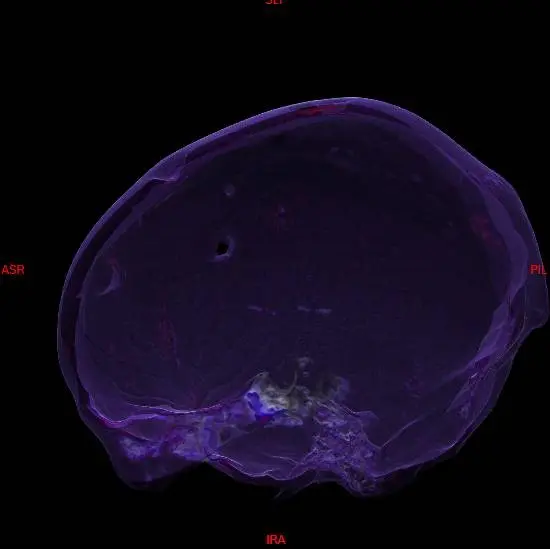Sample code for general purpose colour/opacity adjustment.

The result might not good good on particular data because this is intended purely for demonstration purposes!

`````` Color[] TF = new Color;
int[] lookup = new int[] {
// Hounsfield value, Red, Green, Blue, Alpha
0,0,0,0,0, // 1-100 = transparent
200,0,0,0,0,
580,255,255,255,50,
660,0,0,255,200,
900,255,0,0,0, // 900 up = transparent
1000,255,255,0,0
};

for (int j = 0; j < lookup.Length; j += 5)
{
for (int ii = 0; ii < lookup[j]; ii++)
{
float p0 = (float)(ii - lookup[j - 5]) / (lookup[j] - lookup[j - 5]);
float p1 = (float)(lookup[j] - ii) / (lookup[j] - lookup[j - 5]);

int r = (int)(lookup[j + 1] * p0 + lookup[j - 4] * p1);
int g = (int)(lookup[j + 2] * p0 + lookup[j - 3] * p1);
int b = (int)(lookup[j + 3] * p0 + lookup[j - 2] * p1);
int a = (int)(lookup[j + 4] * p0 + lookup[j - 1] * p1);

TF[ii] = Color.FromArgb(a, r, g, b);
}
}

image_3D.SetTransferFunction(0, 1000, TF); // image_3D is a DicomImage3D instance
``````

Sample output: